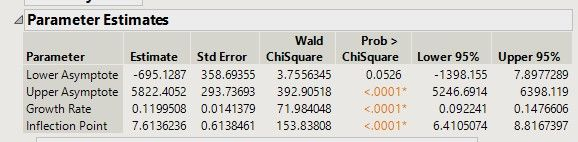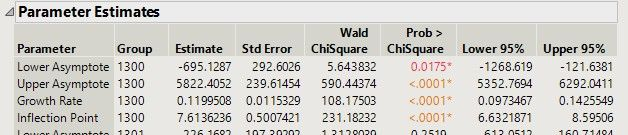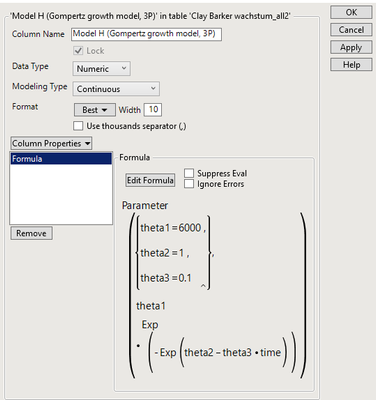Choose Language Hide Translation Bar
Highlighted

## nichtlineare Regression

Darf ich eine nichtlineare Regression für 1 Tier berechnen.

Normalerweise berechne ich dies für mehreren Tieren.

Es soll eine bestimmte Zahl an Tieren am Leben bleiben.

Wenn ich für jedes Tier die nicht lineare Regression nach Gompertz berechne, erhalte ich für jedes Tier den Wendepunkt. Anhand des Wendepunktes soll entschieden werden welches Tier am Leben bleibt. Ist diese Überlegung so richtig?

3 ACCEPTED SOLUTIONS

Accepted Solutions
Highlighted

## Re: nichtlineare Regression

Looking at an individual animal is definitely a reasonable thing to do. The curve’s parameter estimates are independent across animals, so you’ll get the same estimates for each animal whether you look at them individually or all at once. The only difference is that when specifying “ID” as a grouping variable, all of the animals contribute to the estimate of the error variance. So standard errors and inferences may be slightly different.

Here is difference when looking at animal 1300 individually and with the other animals…

These are the results when looking at animal 1300 by itself.And here are the results for animal 1300 when all of the animals are included in the analysis. Notice that the standard errors are slightly lower, which leads to smaller p-values and tighter confidence intervals.The decision comes down to whether or not the animals are expected to share a common error variance. If so, it’s probably better to look at them all at once. But if that assumption doesn’t make sense, it would be better to look at them individually.

Highlighted

## Re: nichtlineare Regression

The starting values are based on the interpretation of the parameter in your model. For example, a simple exponential decay model might be Y = A*Exp(B*time). I would use the mean of Y at time = 0 for A and the difference in Y between the first two times divided by the difference in time for B.

I suggest that you first read Help > Books > Predictive and Specialized Modeling. In particular, see the chapters devoted to the Nonlinear and Fit Curve platforms. They have been written to explain a lot and answer your questions.

Learn it once, use it forever!
Highlighted

## Re: nichtlineare Regression

Yes, Fit Curve and Nonlinear are both for fitting non-linear models, but Fit Curve automates much of the fitting while Nonlinear requires that you specify everything. Since the Gompertz model is available in the Fit Curve platform, I would use it for convenience.

Use the Group analysis role when you launch Fit Curve to get a separate fit for each ID.

Learn it once, use it forever!
11 REPLIES 11
Highlighted

## Re: nichtlineare Regression

Looking at an individual animal is definitely a reasonable thing to do. The curve’s parameter estimates are independent across animals, so you’ll get the same estimates for each animal whether you look at them individually or all at once. The only difference is that when specifying “ID” as a grouping variable, all of the animals contribute to the estimate of the error variance. So standard errors and inferences may be slightly different.

Here is difference when looking at animal 1300 individually and with the other animals…

These are the results when looking at animal 1300 by itself.And here are the results for animal 1300 when all of the animals are included in the analysis. Notice that the standard errors are slightly lower, which leads to smaller p-values and tighter confidence intervals.The decision comes down to whether or not the animals are expected to share a common error variance. If so, it’s probably better to look at them all at once. But if that assumption doesn’t make sense, it would be better to look at them individually.

Highlighted

## Re: nichtlineare Regression

Hello, Mr. Barker,

I can understand all that well.
Now I have calculated the nonlinear regression over all IDs and with by ID.
What I don't like is the line in the plot...

Did I do something wrong?
Should I change something in Preferences?

Thank you very much for your help!

Highlighted

## Re: nichtlineare Regression

Hey,

The nonlinear platform is very powerful, but it is very sensitive to the starting values for each parameter in the model. They are specified in the column formula and I changed the starting values in your column as below:I've attached a modified version of your table with the updated starting values. Now the models should converge to a much more reasonable fit for both of the fits in your scripts.

The Fit Curve platform is nice because it handles things like starting values automatically, but it does use a slightly different parameterization than what you have in you Gompertz model column formula.

Highlighted

## Re: nichtlineare Regression

Hello,

Thank you so much for the answer.
How did you get the starting values. Or how do I get them with a new calculation of the nonlinear regression?
What is the difference between Fit Curve and nonlinear regression?

Thanks!

Highlighted

## Re: nichtlineare Regression

The starting values are based on the interpretation of the parameter in your model. For example, a simple exponential decay model might be Y = A*Exp(B*time). I would use the mean of Y at time = 0 for A and the difference in Y between the first two times divided by the difference in time for B.

I suggest that you first read Help > Books > Predictive and Specialized Modeling. In particular, see the chapters devoted to the Nonlinear and Fit Curve platforms. They have been written to explain a lot and answer your questions.

Learn it once, use it forever!
Highlighted

## Re: nichtlineare Regression

Hello,
you've been working on a totally different formula.
i work with Gompertz 3P =a+Exp(-Exp(-b*(time-c)))
I had looked at the explanation in Help over the Internet.
Even if I calculate with the same data in "Fit Curve" as follows the line in the plot is not ok in my eyes.

``````Fit Curve(
Y( :Y ),
X( :time ),
Group( :ID ),
Fit Gompertz 3P,
SendToReport( Dispatch( {"Gompertz 3P"}, "Parameter Estimates", OutlineBox, {Close( 0 )} ) )
)``````

Why is the line in the plot wrong?

Thanks!

Highlighted

## Re: nichtlineare Regression

I was merely illuistrating the thought process with a common example (simple exponential decay). I was not trying to explain your model parameters (Gompertz). You selected the model so you should be able to interpret the parameters yourself. Do you undestand how to use the interpretation to get a starting value?

Can you explain better what you mean by "the line in the plot is not ok in my eyes?" What specificially are your referring to in the curve? Can you provide a picture of the plot with the incorrect curve?

Are there any data problems or outliers that might affect the fit of the curve?

Learn it once, use it forever!
Highlighted

Hello,

Best regards.

Highlighted

## Re: nichtlineare Regression

Thank you for the document with your results. Unfortunately, you are trying a lot of different things in a haphazard way. Let's start over.

Pick a model. You selected the Gompertz model, so stick with it and get it to work.

Select starting values. What is your interpretation of the three parameters, theta1 to theta3? What do they represent in the data or the change in the data? What are reasonable values based on your data?

Edit the model. Change the current values of the parameters in the model formula to use the selected starting values. I can help after I know what you want to use.

Fit the model. You seem to know how to fit the model with Nonlinear.

Note 1: you can vary the starting parameters in Nonlinear before you click Go! This way is fast and the plot tells you if you are making progress.

Note 2: you can save this example as a template and use it in the future with new data.

Learn it once, use it forever!
Article Labels

There are no labels assigned to this post.